Mobile QR Code1. (School of Computer and Communication Engineering, Changsha University of Science and Technology, Changsha 410114, HN, China)
2. (School of Automotive and Mechanical Engineering, Changsha University of Science and Technology, Changsha 410114, HN, China)

Failure probability, cuckoo algorithm, sensitivity vector, logical circuit, semiconductors

## I. INTRODUCTION

In recent years, As the feature size of Complementary Metal Oxide Semiconductor (CMOS) has shrunk to the nanometer scale, the device performance has been greatly improved, At the same time, however, new problems in circuit design have emerged, such as parameter fluctuations and soft errors , which significantly affect predictability and reliability [2-5]. In particular, soft errors caused by high-energy particle radiation will aggravate the chip failure introduce and great challenges to the process of designing reliable circuits [6,7].

The term "sensitive vector" refers to an input vector that leads to high failure probability in the input excitation space of the circuit. Searching for sensitive vectors first requires accurate estimation of the Failure Probability of Circuits (FPC). Present failure probability estimation methods of logic circuits can be divided into two categories: simulation methods and probability analysis methods. In simulation methods, the faults are randomly injected into the logic element of the circuit, after which the output values are derived via circuit simulation. Finally, the FPC is obtained through comparison with the outputs of the fault-free circuit. A typical simulation method is the Monte Carlo (MC) simulation, MC simulation requires a large number of simulations to obtain accurate results. By contrast, probability analysis methods are orders of magnitude faster than MC simulations. Common probability analysis methods include Probability Transition Matrix (PTM) , Probabilistic Gate Models (PGM) , Critical Score Algorithm (CSA) , etc. Among them, PTM model is an accurate means of calculating the FPC. This method uses the probability transfer matrix of basic logic gates or sub-circuits to estimate the overall FPC. However, its disadvantage is that it requires a lot of storage space during matrix operation, meaning that it is only suitable for small and medium-scale circuits. Compared with the PTM model, the PGM method has linear space complexity, but the exponential time complexity of the fan-out reconvergence cannot be effectively solved. CSA can be used to quickly estimate the FPC corresponding to a specific input vector. Its complexity is linearly related to the circuit size, but the accuracy is inadequate because it does not consider the correlation between the signals.

Selective fault tolerance and hardening of sensitive logic gates corresponding to sensitive vectors will efficiently reduce the failure probability of the circuit and increase the reliability of the system. Sensitive logic gates are gate units that have a direct impact on the failure probability at the output of a circuit, which is related to the input excitation vector and the circuit structure .  proposed Critical Gates Count algorithms (CGC) for verifying whether gate failures affect the circuit. Low-cost fault-tolerant designs can be further aided by locating sensitive logic gates [12,13]. Whether a logic gate is sensitive or not is closely related to the input vector. In addition, different input vectors correspond to different sets of sensitive gates. Searching for sensitive vectors plays an important role in improving circuit reliability [10,14]. When excited by sensitive vectors, the failure probability of some circuits may be several orders of magnitude higher than the average. If these sensitive vectors can be avoided effectively, or the fault tolerance and reinforcement of the circuit can be targeted, the FPC can be reduced with minimum cost. For small-scale circuits, the FPC corresponding to all vectors can be calculated exhaustively due to the relatively small number of inputs. However, for large-scale or even very large-scale circuits, the search vector space increases exponentially with increasing circuit size and number of inputs, meaning that the calculation of the FPC under single-vector excitation becomes more difficult. To solve this problem,  proposed heuristic algorithms to locate sensitive vectors within the vector space. This work employed the Hill Climbing (HC) algorithm, Simulated Annealing (SA) algorithm, Genetic Algorithm (GA) and the extended algorithm of them. However, these algorithms are all prone to falling into local optima, and the search efficiency is inadequate. In , the HC algorithm was used to determine the logic values of some input bits in advance to reduce the search space, and was then combined with the exhaustive method for calculation.  proposed a PTM-based iterative algorithm to search sensitive vectors by using the iterative strategy of MC. However, the accuracy of the search methods mentioned in  and  needs to be improved. In , a method based on gate-sensitive attributes was proposed to estimate the criticality of the primary input leads in combinational circuits with respect to their reliability, and a parallel strategy based on sub-circuits was combined with an adaptive convergence check method to speed up the computation.

In the late 20th century, various heuristic algorithms continued to emerge, including the ant colony algorithm , particle swarm algorithm, etc. These algorithms were inspired by the laws of nature and solved the problem of objective optimization by simulating various behaviors and phenomena exhibited by animals in natural environments. The Cuckoo Search (CS)  algorithm was proposed by Cambridge University scholars Xin-She Yang and Suash Deb in 2009. This global search algorithm was inspired by simulating the behavior of cuckoos searching for nests. A large number of scholars have studied the CS algorithm and proposed improved versions.  proposed a hybrid multi-objective control algorithm, which combines the multi-objective control algorithm, non-dominated sorting algorithm and reference point strategy to ensure the convergence and diversity of the algorithm.  improved the CS algorithm's convergence speed and optimization ability through adaptive adjustment of the discovery probability. In  three new learning strategies for improving algorithm performance observing cuckoo’s nest seeking behavior, expulsion behavior and begging behavior.

In this paper, we use the Correlation Separation Approach (COSEA) to efficiently calculate the FPC, and further propose an Improved Adaptive Cuckoo Search (IACS) algorithm to find the sensitive vector of the circuit. The IACS algorithm uses a vector segmentation strategy to process the input vector, employs the hill climbing algorithm to search the sensitive vector, then introduces an adaptive strategy to dynamically adjust the discovery probability $\textit{P}$$_{a}, power law index {\beta}, and scaling factor \textit{r}. In this way the sensitive vectors can be located quickly and accurately. The remainder of this paper is organized as follows. Section 2 introduces the COSEA for efficiently calculating the FPC under specific vector excitation. Section 3 briefly introduces the basic cuckoo algorithm, after which the fourth section introduces the improved adaptive cuckoo algorithm. Section 5 presents the experimental results. Finally, the sixth section concludes this paper. ## II. CORRELATION SEPARATION APPROACH COSEA is a probability analysis-based approach that fully considers the fan-out reconvergence structure of the circuit. By dividing the circuit into Independent Circuit Structures (ICS), the correlation between the signals of each node is separated, such that the FPC can be calculated accurately. In this paper, the Error Probability of Nodes (EPN) is used to represent the error probability of circuit nodes, while the Fault Probability of Gates (FPG) is used to express the probability that a logic gate will fail. ### 1. Independent Circuit Structures For any target node \textit{i} of the circuit, the error probability can be regarded as the accumulation of error effects of the upstream circuit of the node (the circuit contained in the input cone of \textit{i}, denoted as INC_{i}). In COSEA, the circuit block is divided into two types. In INC_{i}, the output is the fanout node, while the input is the fanout node or the gate node of the original input. The circuit combination is called Fanout-Relevant Circuits (C_{\mathrm{FR}}). Its Failure Probability of C_{\mathrm{FR}} (PFR) fans out to other circuits through the fanout source node, as shown in Fig. 1(a); some circuit module errors do not affect the fanout source node in INC_{i}. The output is the desired target node (non-fanout node), while the input is the fanout node or the original input. This circuit combination is called Fanout-Irrelevant Circuits (C_{\mathrm{FI}}). Its Failure Probability of C_{\mathrm{FI}} (PFI) is as shown in Fig. 1(b). ##### Fig. 1. (a) Fanout-relevant circuits, (b) Fanout-irrelevant circuits.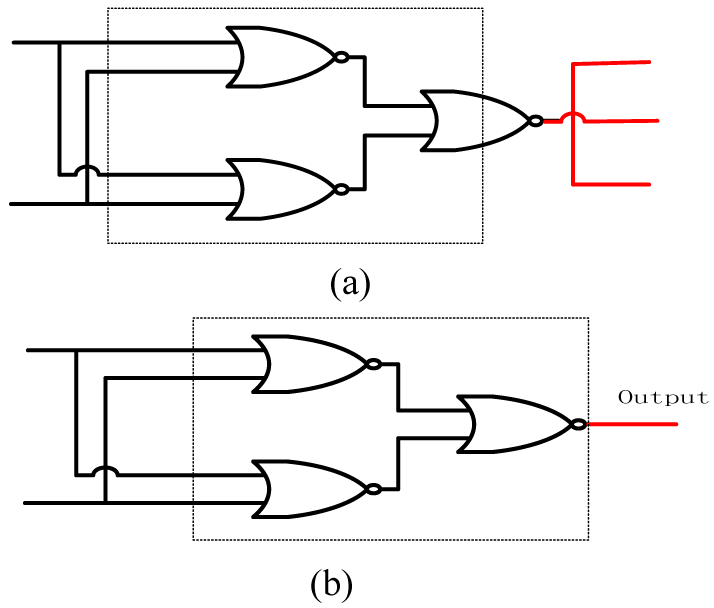### 2. Algorithm Flow The method first extracts the circuit information from the netlist file, determines the logic value of the input signal and the failure probability of the gate, then stores and updates three pieces of information at each node: the logic value LV of the node, the failure probability PFI of the C_{\mathrm{FI}}, and the set U of the C_{\mathrm{FR}}. Based on the information at each node, we can calculate the failure probability of the node as follows: \begin{equation} ##### (1) \mathrm{EPN}_{i}=\mathrm{PFI}_{i}+\sum _{j=1}^{\mathrm{n}}\mathrm{PFR}_{j} where EPN_{i} denotes the failure probability of node \textit{i}, n denotes the number of elements in the set of C_{\mathrm{FR}}, PFR_{j} denotes the failure probability of fanout-relevant circuits C_{\mathrm{FR}}$$_{j}$, and PFI$_{i}$ denotes the failure probability of fanout-irrelevant circuits C$_{\mathrm{FI}}$$_{i}. For node \textit{i}, the logical value of its input signal determines which C_{\mathrm{FR}} and C_{\mathrm{FI}} on the input signal can affect the output result; thus, the C_{\mathrm{FR}}, C_{\mathrm{FI}} and logical values of the input signal need to be determined in order to calculate the node failure probability. There may be more than one C_{\mathrm{FR}} affecting a node, and the set of all C_{\mathrm{FR}} affecting a node is denoted by U. Moreover, the is at most one C_{\mathrm{FI}} affecting a node; thus, we only need to calculate the PFI of the failure probability of the C_{\mathrm{FI}} corresponding to each node. The failure probability of C_{\mathrm{FR}} is not calculated directly, but is instead obtained by converting C_{\mathrm{FI}} to C_{\mathrm{FR}}, as illustrated in Fig. 2. Here, nodes \textit{a}, \textit{b} and \textit{c} represent the logic gates in the circuit, while the lines represent the connections in the circuit. When calculating the failure probability at point \textit{a}, C_{\mathrm{FI(}}$$_{a}$$_{\mathrm{)}} is the C_{\mathrm{FI}} with point \textit{a} as the output; when the calculation reaches point \textit{b}, the range of C_{\mathrm{FI(}}$$_{b}$$_{\mathrm{)}} includes C_{\mathrm{FI(}}$$_{a}$$_{\mathrm{)}} and point \textit{b}. As point \textit{b} is \textit{a} fanout node, we calculate the failure probability of C_{\mathrm{FI(}}$$_{b}$$_{\mathrm{)}} fanout at point \textit{b}, at which point C_{\mathrm{FI(}}$$_{b}$$_{\mathrm{)}} is converted to C_{\mathrm{FR(}}$$_{b}$$_{\mathrm{)}}. As shown in Fig. 2(c), the failure probability PFR of the converted C_{\mathrm{FR}} is equal to the failure probability of the PFI before conversion, while the PFI indicated by this node is set to 0. ##### (2) \begin{equation} \left\{\begin{array}{l} \mathrm{C}_{\mathrm{FR}\left(b\right)}\leftarrow \mathrm{C}_{\mathrm{FI}\left(b\right)}\\ \mathrm{C}_{\mathrm{FI}\left(b\right)}\leftarrow \varnothing \end{array}\right.,\left\{\begin{array}{l} \mathrm{PFR}_{\left(b\right)}=\mathrm{PFI}_{\left(b\right)}\\ \mathrm{PFI}_{\left(b\right)}=0 \end{array}\right. \end{equation} The new C_{\mathrm{FI(}}$$_{b}$$_{\mathrm{)}} no longer represents the range of the original C_{\mathrm{FI(}}$$_{b}$$_{\mathrm{)}}, but rather the C_{\mathrm{FI}} circuit starting with the output of \textit{b}, without including any nodes. Thus, the new C_{\mathrm{FI(}}$$_{b}$$_{\mathrm{)}} is the empty set, which corresponds to the failure probability PFI_{\mathrm{(}}$$_{b}$$_{\mathrm{)}} = 0. ##### Fig. 2. The process of converting C_{\mathrm{FI}} to C_{\mathrm{FR}}.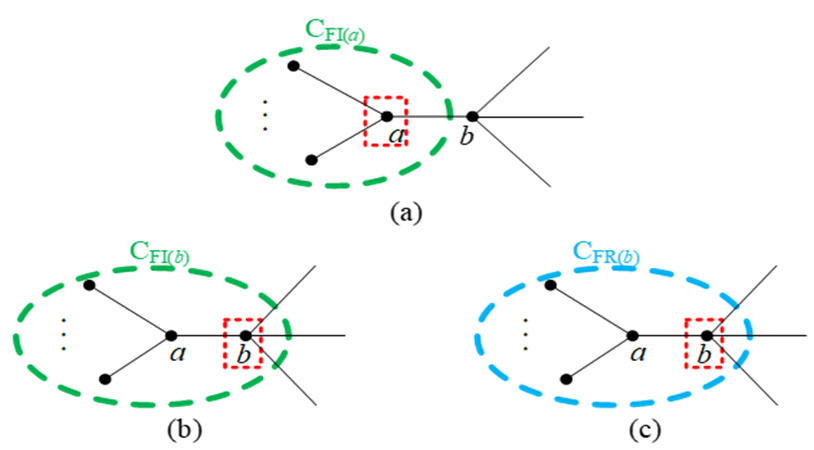The failure probability of the circuit is accurately calculated based on the failure probability of the fanout-irrelevant node, the failure probability of each parent node and the logic value of the parent node iterated through to the output of the circuit. If the circuit under evaluation circuit is a single-output circuit, then the FPC is the original output node error rate. Otherwise, we first assume that the circuit contains \textit{m} original outputs; since the error rate of each output node is composed of PFI and PFR, and the PFIs of any two output nodes are independent of each other while the different PFRs are also independent of each other, the failure probability of a multi-output circuit can be expressed as follows: ##### (3) \mathrm{FPC}=1-\prod _{i=1}^{m}\left(1-\mathrm{PFI}_{i}\right)\times \prod _{j=1}^{n}（1-\mathrm{PFR}_{\mathrm{j}}） where \textit{n} denotes the number of elements contained in the concatenated set of all original output nodes that fanout to the source set. The algorithm about the calculation of COSEA are presented in Fig. 3. ##### Fig. 3. Pseudo-code of the COSEA algorithm.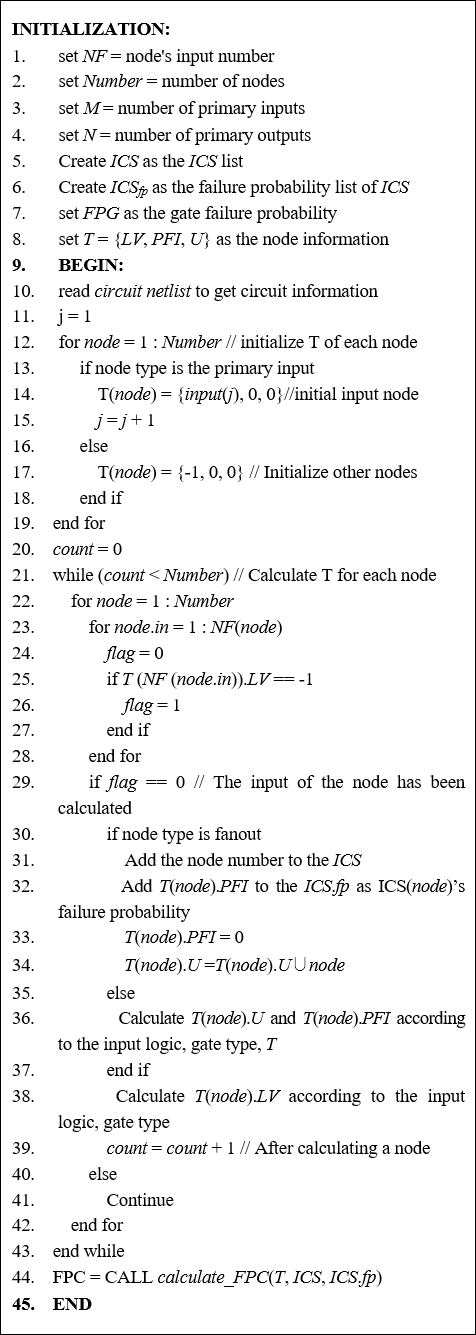## III. BASIC CUCKOO ALGORITHM In nature, cuckoos use parasitic brood rearing to produce offspring. Specifically, they surreptitiously place their eggs in the nests of their hosts and allow them to incubate their offspring. If the foreign eggs are discovered by the host, the host will discard them. Rule 1: The cuckoo lays one egg at a time and chooses a random nest location for incubation. Rule 2: Of the randomly selected nests, the best nest is chosen to be kept for the next generation. Rule 3: The number of available nests is fixed and the probability that an incoming egg will be found in a nest is \textit{P}$$_{a}$, ($\textit{P}$$_{a} {\in} [0,1]). Based on the above rules, the algorithm about the calculation of CS is presented in Fig. 4. ##### Fig. 4. Pseudo-code of the CS algorithm.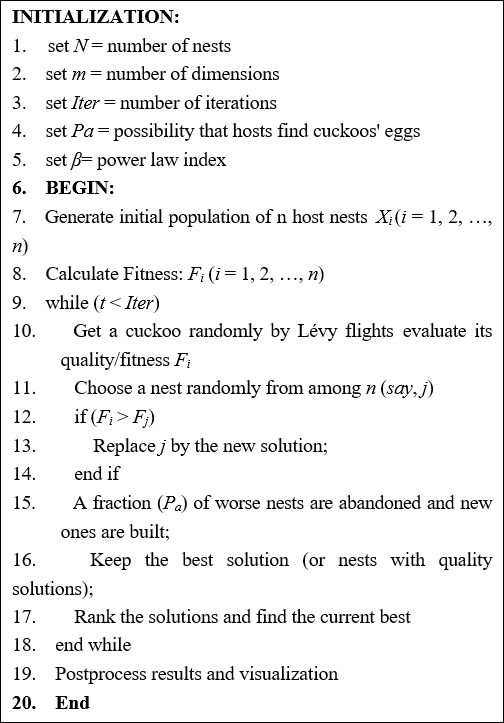The cuckoo algorithm searches for the path and location of the bird's nest by L\'{e}vy flight random tour, as shown in Eqs. (4) and (5): ##### (4) X_{i}^{t} =X_{i}^{t-1}+\alpha \oplus L\text{é}vy(\lambda ) \\ ##### (5) X_{i}^{t} =X_{i}^{t-1}+r(X_{j}^{t-1}-X_{k}^{t-1}) In Eqs. (4) and (5), X_{i}^{t} and X_{i}^{t-1} represent the nest positions at generation \textit{t} and \textit{t}-1, respectively, {\alpha} is the step control factor, the symbol {\oplus} denotes the point-to-point multiplication, and L\text{évy}(\lambda ) represents the L\text{évy} random search path; moreover, \textit{r} represents the scaling factor, which is a random number uniformly distributed in the interval [0, 1] for scaling control, while \textit{X}$$_{j}$ and $\textit{X}$$_{k} denote the two random solutions at generation \textit{t}-1. L\text{évy}(\lambda ) follows the L\text{évy} probability distribution, as shown in Eq. (6): ##### (6) L\text{é}vy\left(\lambda \right)\sim u=t^{-\lambda },（1<\lambda \leq 3） Eq. (6) shows that the successive position changes of the cuckoo are a random walk that obeys a power-law distribution. One of the L\text{évy} flight random search path formulas is presented in Eq. (7): ##### (7) \text{Step}=\frac{\mu }{\left| v\right| ^{\frac{1}{\beta }}} Step'' in Eq. (7) is the L\text{évy} random search path for L\text{évy}(\lambda ) in Eq. (4), and the power law exponent {\beta} is related to {\lambda} in Eq. (6); specifically, {\lambda} = 1 + {\beta}, where {\beta} {\in} (0, 2]. The parameters \mu and \nu both obey a normal distribution, i.e., \mu \sim N(0,\sigma _{\mu }^{2}),\nu \sim N(0,1), where: ##### (8) \sigma _{\mu }=\left\{\frac{\Gamma \left(1+\beta \right)\sin \left(\pi \beta /2\right)}{\Gamma \left[\left(1+\beta \right)/2\right]\beta 2^{\left(\beta -1\right)/2}}\right\}^{1/\beta } ## IV. IMPROVED ADAPTIVE CUCKOO ALGORITHM AND SENSITIVE VECTOR SEARCH ### 1. Adaptive Strategies In the basic cuckoo algorithm, parameters such as the power law exponent {\beta}, discovery probability \textit{P}$$_{a}$ and scaling factor $\textit{r}$ are introduced. The settings of these parameters will have a great impact on the convergence of the global search and local search of the algorithm; thus, the parameter setting needs to balance the global search and convergence. In the basic CS algorithm, these parameters are fixed values, which is not a situation conducive to striking a balance between the algorithm's global and local random search. To overcome these shortcomings, we introduce an adaptive strategy to adjust these parameters with the goal of improving the solution search accuracy.

(1) Adaptive strategy with power-law exponent ${\beta}$

Fig. 5 and 6 show the fluctuation of the step at different values of ${\beta}$. From the figures, it can be seen that when ${\beta}$ is small, the fluctuation range of the step size is too large, which is not conducive to the convergence of the solution; when ${\beta}$ is large, moreover, the fluctuation range of the step size is too small, which is not conducive to the global search for the solution. Therefore, we set ${\beta}$ to fall within the range of [0.8,1.8] and use the adaptive strategy.

The values of ${\beta}$ are shown in Eqs. (9) and (10) below:

##### (9)
$l_{i} =1-\frac{\left\| n_{i}-n_{best}\right\| }{l_{\max }} \\$
##### (10)
$\beta _{i} =\beta _{\min }+(\beta _{\max }-\beta _{\min })l_{i}$

In Eq. (9), $\textit{n}$$_{i} denotes the current nest position, \textit{n}$$_{best}$ denotes the optimal nest position at this time, and $\textit{l}$$_{max} denotes the maximum distance between the optimal position and the remainder of the nest positions. In Eq. (10), {\beta}$$_{max}$ denotes the maximum value of ${\beta}$ (${\beta}$$_{max}=1.8), while {\beta}$$_{min}$ denotes the minimum value of ${\beta}$ (${\beta}$$_{min}=0.8). This adaptive strategy indicates that if the current nest is close to the optimal nest position, the fluctuation of its step size will be reduced; conversely, if the current nest is far from the optimal nest position, the fluctuation of its step size will be increased to facilitate a better search for the optimal solution. (2) Discovering adaptive strategies for probabilistic \textit{P}$$_{a}$

##### (14)
$P_{a\_ i}^{t}=P_{a\_ \min }+\left(P_{a\_ \max }-P_{a\_ \min }\right)\left(1-\frac{f_{i}^{t}}{f_{best}^{t}}\right)$

##### Table 2 Statistical results for different $\textit{P}$$_{a} values  Function \textit{P}$$_{a}$=0.01 $\textit{P}$$_{a}=0.1 \textit{P}$$_{a}$=0.2 $\textit{P}$$_{a}=0.3 \textit{P}$$_{a}$=0.4 $\textit{P}$$_{a}=0.5 \textit{P}$$_{a}$=0.6 $\textit{P}$$_{a}=0.7 \textit{P}$$_{a}$=0.8 $\textit{P}$$_{a}=0.9 \textit{P}$$_{a}$=1 $f_{1}$ 3.7e-7 7.2e-9 8.3e-9 4.5e-9 1.1e-9 2.9e-10 1.8e-11 3.7e-14 2.1e-12 9.3e-11 8.2e-11 $f_{2}$ 1.3 9e-1 2.4e-1 4.2e-1 2.8e-1 3.3e-1 4.8e-1 4.6e-1 3.5e-1 5e-1 6.6e-1 $f_{3}$ 3.9e-1 1.8e-1 5e-2 2.2e-2 0.1e-1 4.8e-3 1.5e-2 3.8e-2 3.9e-2 5.7e-2 6.3e-2

(3) Adaptive strategy for scaling factor $\textit{r}$

In the basic cuckoo algorithm, the scaling factor $\textit{r}$ is a uniformly distributed random number in the interval [0, 1]. Its randomness does not guarantee a large variety of the worse individuals. If the scaling factor $\textit{r}$ can be better controlled to produce a large variety of the worse individuals and thus improve the algorithm's solution accuracy, this paper uses an adaptive mechanism to control the scaling factor r with the value interval [0, 1].

The scaling factor $\textit{r'}$s adaptive strategy can be expressed as follows:

##### (15)
$r_{i}^{t}=r_{\min }+\left(r_{\max }-r_{\min }\right)\frac{f_{i}^{t}-f_{best}^{t}}{f_{\textit{worst}}^{t}-f_{best}^{t}}$

In Eq. (15), $r_{i}^{t}$ denotes the scaling factor of the $\textit{i}$th individual in the $\textit{t}$th generation population, $\textit{r}$$_{\mathrm{max}} and \textit{r}$$_{\mathrm{min}}$ denote the upper and lower bounds of the scaling factor, and $f_{i}^{t}$, $f_{best}^{t}$ and $f_{\textit{worst}}^{t}$ denote the fitness of the $\textit{i}$th, best and worst individuals in the $\textit{t}$th generation population, respectively. This means that the further the current nest is from the optimal nest, the larger the $\textit{r}$-value, and the further farther the updated nest is from the current nest; conversely, the closer the optimal nest location, the smaller the $\textit{r}$-value, and the closer the updated nest is to the current nest.

### 2. Improving Initial Population Quality through Hill Climbing Algorithms

In the basic cuckoo algorithm, the initial population is randomly selected, meaning that the initial population is of poor quality. To solve this problem, we replace the randomly selected initial population with a new population that has been optimized using the hill-climbing algorithm. The quality of the new population is superior to the quality of the randomly selected initial population used to optimize the cuckoo algorithm. We introduce the concept of adjacency, where input vectors that differ by one bit are considered to be adjacent. The climbing algorithm works by finding the vectors adjacent to the input vector, comparing them, saving the adjacent vector if it is better, and repeating the process until the iteration condition is met. The final better solution is found by taking the first $\textit{n}$ better solutions as the initial population according to the number of nests $\textit{n}$.

The algorithm for initializing population calculation of HC algorithm is presented in Fig. 10.

##### Fig. 10. Pseudo-code of the Hill Climbing (HC) algorithm initialization population.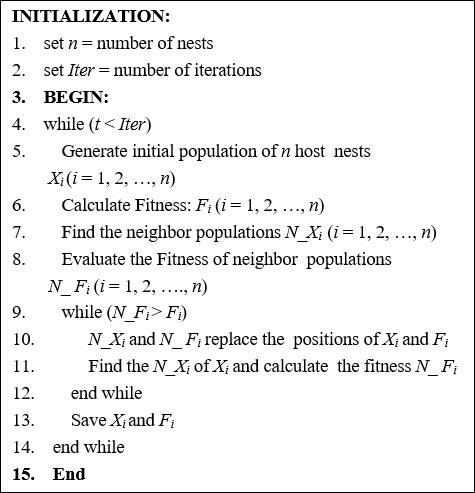### 3. Input Vector Segmentation

The input vector to the logic circuit is a sequence of consecutive binary codes. To facilitate the calculation, we convert the binary to decimal numbers, which are then used as input to the cuckoo algorithm. However, as the number of inputs to the circuit increases, any binary numbers that are too long cannot be converted to decimal numbers. We therefore propose a vector partitioning method to improve the cuckoo algorithm's search capability by dividing the longer binary numbers into several segments and reducing the vector space to be searched in each segment.

1.Determine the number of bits of the input vector $\textit{num}$;

2.Divide the input vector into equal segments, such that the number of bits in each segment is $\textit{c}$;

3.Convert each segment of the partitioned binary number to a decimal number;

4.Organize the converted decimal numbers into a mapping set $\textit{T}$;

5.Determine the upper and lower bounds as $[0,\sum _{j=0}^{c-1}2^{j}]$.

The conversion equations are presented in Eqs. (16)-(18):

##### (16)
$n =\left\lceil num/c\right\rceil ,0<c\leq num \\$
##### (17)
$Int(b_{i}) =\sum _{j=0}^{k-1}a_{ij}\times 2^{j},k\leq c,i\in [1,2,3,\ldots ,n] \\$
##### (18)
$T =\left[Int(b_{1}),Int(b_{2}),\ldots ,Int(b_{i})\right]$

Here, $\left\lceil \right\rceil$ represents rounding up, $\textit{b}$$_{i} stands for the \textit{i}th fragment, and Int(\textit{b}$$_{i}$) denotes mapping binary $\textit{b}$$_{i} to decimal. In the vector partitioning method, \textit{num} may not be an integer multiple of \textit{c}; this results in the last segment of the vector being of length \textit{r}(0<\textit{r}<\textit{c}), where the vector of length \textit{r} is still converted to decimal, corresponding to its range bound of [0,\sum _{j=0}^{r-1}2^{j}]. For example, suppose that the input vectors of circuit 1 and circuit 2 are '100010001111' (12 bits) and '10001000111' (11 bits) respectively, and that \textit{c}=3. In this case, the above analysis shows that the mapping set \textit{T} of vector 1 is [4, 2, 1, 7], the mapping set \textit{T} of vector 2 is [4, 2, 1, 3], the lower bound of circuit 1 is [0, 0, 0, 0] and the upper bound is [7, 7, 7, 7], while the lower bound of circuit 2 is [0, 0, 0, 0] and the upper bound is [7, 7, 7, 3]. Based on the above analysis, the IACS algorithm searches for sensitive vectors as shown in Fig. 11. ##### Fig. 11. Pseudo-code of the IACS algorithm.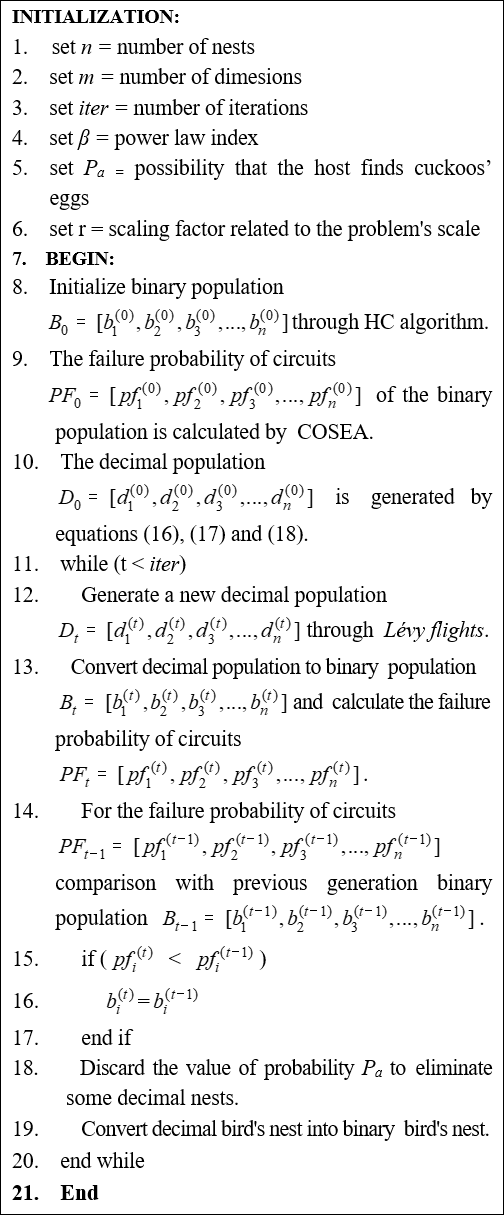## V. EXPERIMENTAL RESULTS AND ANALYSIS In this section, in order to evaluate the effectiveness of the proposed improved adaptive cuckoo algorithm for searching circuit sensitive vectors, we conduct the following analysis: 1. COSEA is compared with other FPC calculation methods; 2. The influence of the algorithm vector partitioning parameter \textit{c} on the search accuracy of the cuckoo algorithm is studied; 3. The proposed method is compared with the hill climbing algorithm, simulated annealing algorithm and genetic algorithm on benchmark circuits of different sizes. The experiments were carried out on a computer with a 2.66 GHz Pentium microprocessor and 2 GB of RAM, on PyCharm and Win10, using Python as the programming language. We use the results of the MC method to calculate the FPC as a reference standard. The average error Error(COSEA) and Error(CSA) of the COSEA method, CSA method and MC method are calculated as follows: ##### (19) \text{Error}(\text{COSEA}) =\frac{1}{n}\times \sum _{i=1}^{n}\left| \frac{\mathrm{FPC}_{\mathrm{MC}(i)}-\mathrm{FPC}_{\text{COSEA}(i)}}{\mathrm{FPC}_{\mathrm{MC}(i)}}\right| \\ ##### (20) \text{Error}(\mathrm{CSA}) =\frac{1}{n}\times \sum _{i=1}^{n}\left| \frac{\mathrm{FPC}_{\mathrm{MC}(i)}-\mathrm{FPC}_{\mathrm{CSA}(i)}}{\mathrm{FPC}_{\mathrm{MC}(i)}}\right| Here, \textit{n} is the number of input vectors calculated for each circuit. \mathrm{FPC}_{\mathrm{MC}(i)}, \mathrm{FPC}_{\text{COSEA}(i)} and \mathrm{FPC}_{\mathrm{CSA}(i)} are the FPC under the \textit{i}th input vector excitation calculated by the MC method, COSEA method and CSA methods, respectively. ### 1. COSEA vs. MC and CSA In order to assess the accuracy of COSEA when calculating the failure probability of large circuits, a comparison with the MC method and the CSA method was conducted. The accuracy of the MC method is related to the number of simulations and requires a large number of simulation runs to be executed in order to obtain stable results. Here, we have 5*10e5 simulations for each vector using the MC method. The input vectors chosen for comparison are all zeros and all ones, and 85 series were chosen. The results of the tests are presented in Table 3. The CSA method can only calculate the failure probability of a single output circuit. Here, the maximum number (10) of sub-circuits of C7552 are chosen (in the below, C7552\_i represents the \textit{i}th output containing the C7552 circuit). We here set FPG=10e-4 and the input vectors to full 0 and full 1. Table 4 provides the specific information for these ten experimental circuits. Fig. 12 plots the relative errors of COSEA and CSA against MC simulations. As can be seen from Table 3, the average error is only 0.7318\%, while the time consumption of COSEA is several orders of magnitude higher than MC. Fig. 12 shows that the CSA algorithm has a maximum error of 118\% and an average error of 34\%; this is largely due to the fact that the CSA method does not take the effect of fanout reconvergence in the logic circuit into account, and is moreover less accurate. The COSEA algorithm has a maximum error of 1.59\% and an average error of 0.8429\%. Our experimental results show that the accuracy of the proposed COSEA algorithm is much higher than that of CSA. ##### Table 3 Performance comparison between COSEA and MC  Circuits Gates Inputs Outputs COSEA MC Error (COSEA) (%) Full 0 Full 1 Time (s) Full 0 Full 1 Time (s) C432 268 36 7 0.009858 0.009363 0.0388 0.009912 0.009228 5418 1.00 C499 826 41 32 0.019019 0.019804 0.1217 0.018972 0.019562 22141 0.742 C880 383 60 26 0.019025 0.012622 0.0429 0.019072 0.012338 6276 1.27 C1355 546 41 32 0.015875 0.016661 0.0897 0.016036 0.016460 9839 1.11 C1908 880 33 25 0.060320 0.045632 0.0907 0.060380 0.045920 12634 0.363 C2670 1193 233 140 0.051913 0.034980 0.1206 0.051076 0.035024 18855 0.882 C3540 1669 50 22 0.049728 0.035369 0.1745 0.049748 0.035064 24812 0.455 C5315 2307 178 123 0.087636 0.057113 0.3092 0.088116 0.057166 37470 0.319 C6288 2416 32 32 0.195569 0.195489 0.4208 0.194880 0.193592 46447 0.509 C7552 3512 207 108 0.133613 0.130486 0.4018 0.132882 0.129468 50584 0.668 Average 1400 91 55 — — 0.1850 — — 23447 0.7318 ##### Table 4 Number of gates and inputs for the C7552 sub-circuit  Circuits Gates Input C7552_86 1096 194 C7552_106 676 94 C7552_73 606 124 C7552_59 600 124 C7552_60 600 124 C7552_105 598 80 C7552_93 500 94 C7552_94 500 94 C7552_77 499 94 C7552_87 498 94 ##### Fig. 12. Failure probability relative error of COSEA and CSA compared with MC simulation of 10 sub-circuits of C7552.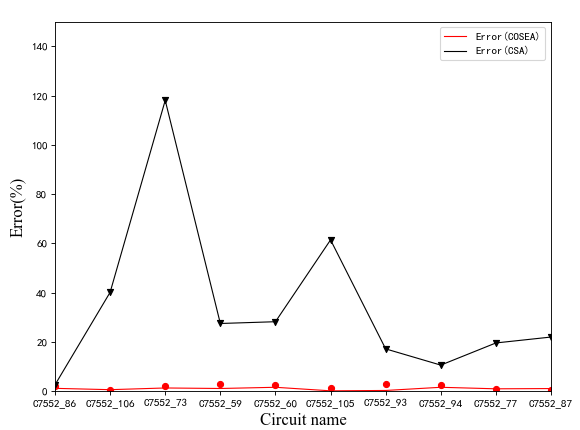### 2. Discussion of the Vector Partitioning Strategy Parameter c We split the long input vector into several segments, then convert each segment to decimal in order to form a new input feature. The value of parameter \textit{c} is too large, which is not conducive to the global search of the algorithm; moreover, due to computational performance limitations, the long binary data cannot be converted into decimal data, meaning that the parameter \textit{c} cannot take too large a value. In summary, the value range of \textit{c} is set from 1 to 5. Experimental results are shown in Table 5. The results are summarized in Table 5, with the best values in bold and the worst values in italics for each row. As the data shows, the results are worse for \textit{c} values of 1 and 5, and better for \textit{c} values of 3 compared to the other values. The results improve when \textit{c} is set to between 1 and 3, while they deteriorate when \textit{c} is set from 3 to 5. In conclusion, setting \textit{c} to 3 produces the best effect in this experiment. ##### Table 5 Experimental results for the values of vector partition n-umber \textit{c}  Circuits Inputs c=1 c=2 c=3 c=4 c=5 FPC_{\mathrm{max}} FPC_{\mathrm{max}} FPC_{\mathrm{max}} FPC_{\mathrm{max}} FPC_{\mathrm{max}} C432 36 0.014598 0.015287 0.015485 0.015287 0.014598 C499 41 0.061724 0.062287 0.062287 0.062287 0.062287 C880 60 0.033924 0.034311 0.034117 0.034021 0.034117 C1355 41 0.042189 0.042380 0.042380 0.042380 0.042380 C1908 33 0.062754 0.063036 0.063130 0.063036 0.062942 C2670 233 0.068177 0.068550 0.076647 0.070440 0.067898 C3540 50 0.070419 0.071164 0.071628 0.070597 0.069854 C5315 178 0.128227 0.131013 0.128488 0.127442 0.125870 C6288 32 0.200782 0.201022 0.201581 0.201102 0.201102 C7552 207 0.189848 0.189524 0.191952 0.189929 0.191467 ### 3. Comparison Between the Method Proposed in This Paper and Other Algorithms In this experiment, each algorithm was run 10 times independently when FPG=10e-4, and the maximum failure probability and running time of the algorithm run results were selected. From the above experimental analysis, we can determine that the number of IACS fixed evolution iterations is 500, the number of hill climbing algorithm iterations is 1000, the number of nests is 5, the upper and lower bounds of probability \textit{P}$$_{a}$ are be 0.2 and 0.8, the upper and lower bounds of the scaling factor $\textit{r}$ are 0 and 1, and the upper and lower bounds of the power law exponent ${\beta}$ are 0.8 and 1.8. We measure the performance of the algorithm by the two indexes of maximum failure probability and time performance. The comparison between the cuckoo algorithm and other algorithms is analyzed below.

In our experiments, the parameters of the hill climbing algorithm, simulated annealing algorithm and genetic algorithm are a crucial element of algorithm effectiveness. For reasons related to time and efficiency, we set the algorithmic parameters as follows; The hill climbing algorithm is a simple local meritocratic method, using heuristics, which is essentially a gradient descent algorithm; the hill climbing algorithm is set to a fixed number of iterations, namely 10000; the simulated annealing algorithm is an optimization algorithm designed based on the concept of the Monte Carlo simulation, which is also essentially a greedy algorithm; the parameters of the simulated annealing algorithm are set to an initial temperature of 1000 and an end temperature of 10e-4. The genetic algorithm is a computational model of biological evolution that simulates the natural selection and genetic mechanism of Darwin's theory of biological evolution, and is widely used in various optimization problems; the parameters of the genetic algorithm are set to 500 for the initial population, 200 for the fixed number of iterations, 0.8 for the crossover rate and 0.1 for the mutation rate; the Randomized Algorithm (RA) randomly selects 100000 input vectors in each circuit, then selects the largest failure probability result among them. The results are presented in Table 6, with the best value marked in bold and the worst value in italics for each row, while "\textemdash{}" indicates that the search time was too long to calculate the results.

##### Table 6 Results of the cuckoo algorithm on finding the maximum failure probability compared to other algorithms
 Circuits Inputs Gates IACS HC SA GA RA FPC$_{\mathrm{max}}$ FPC$_{\mathrm{max}}$ FPC$_{\mathrm{max}}$ FPC$_{\mathrm{max}}$ FPC$_{\mathrm{max}}$ C432 36 619 0.015485 0.014499 0.015485 0.015287 0.014006 C499 41 1571 0.062287 0.061536 0.061348 0.060784 0.061348 C880 60 906 0.034117 0.033634 0.032860 0.033828 0.033248 C1355 41 1387 0.042380 0.042093 0.041614 0.041518 0.041901 C1908 33 1933 0.063130 0.062661 0.062661 0.062942 0.062004 C2670 233 2810 0.076647 0.065002 0.066031 0.064909 0.065562 C3540 50 3562 0.070597 0.067625 0.068930 0.068550 0.067802 C5315 178 5438 0.128227 0.119990 0.116638 0.120781 0.119638 C6288 32 6320 0.201581 0.199742 0.198781 0.196695 0.199422 C7552 207 7660 0.189848 0.186438 — 0.185543 0.185216 S38417 1664 40081 0.787572 0.776290 — — — S38584 1464 40162 0.723219 0.708370 — — — b21 522 44630 0.450985 0.414846 — — — b22 767 65151 0.550538 0.519070 — — —

From the test results in Table 6, it can be seen that the cuckoo algorithm is significantly better at finding the maximum failure probability than the other four algorithms.

To compare the time performance of the algorithms, the parameters of the algorithm were designed as described above. The time of maximum failure probability was determined from Table 6 and shown in Table 7.

The main time consuming aspect of the algorithms is the calculation of the failure probability of the circuits with different input vectors, and the number of vectors calculated is mainly related to the number of iterations of the algorithm and the size of the population. The number of vectors needed to be calculated by the cuckoo algorithm is much lower than that of the three compared algorithms without losing accuracy. Assuming that the circuit failure probability time is calculated as $\textit{t}$($\textit{pf}$) for a single input vector, the time to optimize the population of the hill-climbing algorithm is $\textit{t}$($\textit{hc}$), the population size is $\textit{N}$, and the maximum number of iterations is $\textit{iter}$, then the complexity of the cuckoo algorithm is $\textit{O}$($\textit{iter}$*$\textit{N}$*$\textit{t}$($\textit{pf}$)+ $\textit{N*t(hc}$)).

Due to the limitations of the brute force search algorithm, we selected some small-scale circuits with less than 20 inputs for the test circuit. Moreover, due to the small size of the test circuit, we set the number of fixed iterations of the hill-climbing algorithm to the same number of nests and left the rest of the parameters unchanged.

The following table presents a comparison of the time performance of the two methods when finding the maximum failure probability of the tested circuit for FPG=10e-4. Table 8 shows the superiority of the cuckoo algorithm in terms of time efficiency for a larger number of circuit inputs.

##### Table 7 Time performance results of the cuckoo algorithm compared with other algorithms
 Circuits Inputs Gates IACS HC SA GA RA time(s) time (s) time (s) time (s) time (s) C432 36 619 285.89 768.54 62216.83 3044.63 3581.70 C499 41 1571 908.46 3288.31 204.62 9842.76 11676.01 C880 60 906 373.54 1345.57 820.76 4835.77 5172.45 C1355 41 1387 636.50 2445.20 138.17 6981.49 9242.91 C1908 33 1933 1034.99 2529.72 25482.01 14143.50 11662.71 C2670 233 2810 1262.84 2757.22 13235.13 14744.12 20120.23 C3540 50 3562 1731.05 4092.66 321231.1 20626.57 25330.59 C5315 178 5438 2786.42 9514.45 1322.58 31516.95 30769.92 C6288 32 6320 3766.71 9479.51 33193.78 42322.73 44916.16 C7552 207 7660 3957.20 8671.25 — 56935.27 47925.75 S38417 1664 40081 54588.54 143007 — — — S38584 1464 40162 52196.59 137032 — — — b21 522 44630 80923.64 279944 — — — b22 767 65151 110176.1 384080 — — —
##### Table 8 Results of the cuckoo algorithm compared to the violent search algorithm
 Circuits Inputs IACS Brute-force search time(s) time(s) 74283 9 2.655 3.045 C181 14 52.2 257.266 S208 18 76.528 2904.089

## VI. CONCLUSION

In this paper, an efficient and accurate COSEA is proposed to calculate the failure probability of a circuit, based on preexisting methods, and an improved adaptive cuckoo algorithm based on the hill climbing algorithm is used to search for sensitive vectors. First, we use the hill climbing algorithm to initialize the population in order to improve the initial population fitness value. We then propose a vector partitioning strategy for the processing of circuit input vectors. Finally, we optimize the search efficiency of the cuckoo algorithm using an adaptive strategy with power law exponent ${\beta}$, discovery probability $\textit{P}$$_{a}$, and scaling factor $\textit{r}$. In our comparative experiments, our approach is found to be superior to the three previously proposed algorithms in terms of accuracy and time; thus, we conclude that the proposed method has a great advantage in searching sensitive vectors.

## ACKNOWLEDGMENTS

This work was supported by the National Natural Science Foundation of China (NSFC) (Grant No. 62172058, No. 61702052), Hunan Provincial Natural Science Foundation of China (Grant No. 2020JJ4622, No. 2020JJ5604), and the Hunan Provincial Innovation Foundation for Postgraduate (Grant No. CX20210810).

## References

1
Borkar S., Nov.-Dec., 2005, Designing reliable systems from unreliable components: the challenges of transistor variability and degradation, IEEE Micro, Vol. 25, No. 6, pp. 10-162
Meindl J. D., Chen Q., Davis J. A., Sep. 2001, Limits on Silicon Nanoelectronics for Terascale Integration, Science, Vol. 293, No. 5537, pp. 2044-20493
Lorenz J. K., et al , Aug. 2011, Hierarchical Simulation of Process Variations and Their Impact on Circuits and Systems: Results, IEEE transactions on electron devices, Vol. 58, No. 8, pp. 2227-22344
Shanbhag N. R., et al , July-Aug. 2008, The Search for Alternative Computational Paradigms, IEEE Design & Test of Computers, Vol. 25, No. 4, pp. 334-3435
Xiao J., Lee W., Jiang J., Yang X., 2016, Circuit reliability estimation based on an iterative PTM model with hybrid coding, Microelectronics Journal, Vol. 52, No. 8, pp. 117-1236
Xiao J., Ma W., Lou J., et al , Dec. 2019, Circuit reliability prediction based on deep autoencoder network, Neurocomputing, Vol. 370, pp. 140-1547
Cai S., He B., Wang W., et al. , 2020, Soft error reliability evaluation of nanoscale logic circuits in the presence of multiple transient faults., Journal of Electronic Testing, Vol. 36, No. 4, pp. 469-4838
Krishnaswamy S., Viamontes G. F., Markov I. L., Hayes J. P., 2005, Accurate reliability evaluation and enhancement via probabilistic transfer matrices, Design, Automation and Test in Europe, Vol. 1, pp. 282-2879
Han J., Chen H., Boykin E., Fortes J., 2011, Reliability evaluation of logic circuits using probabilistic gate models, Microelectronics Reliability, Vol. 51, No. 2, pp. 468-47610
Ibrahim W., Shousha M., Chinneck J. W., May. 2015, Accurate and Efficient Estimation of Logic Circuits Reliability Bounds, IEEE Transactions on Computers, Vol. 64, No. 5, pp. 1217-122911
Ibrahim W., Ibrahim H., 2018, Multithreaded and Reconvergent Aware Algorithms for Accurate Digital Circuits Reliability Estimation, IEEE Transactions on Reliability, Vol. 68, No. 2, pp. 514-52512
Xiao J., Shi Z., Zhu W., et al , 2020, Uniform non-Bernoulli sequences oriented locating method for reliability-critical gates, Tsinghua Science and Technology, Vol. 26, No. 1, pp. 24-3513
Xiao J., Shi Z., Yang X., et al , 2021, BM-RCGL: Benchmarking Approach for Localization of Reliability-Critical Gates in Combinational Logic Circuits., IEEE Transactions on Computers14
Ibrahim W., June. 2015, Identifying the Worst Reliability Input Vectors and the Associated Critical Logic Gates, IEEE Transactions on Computers, Vol. 65, No. 6, pp. 1748-176015
Xiao J., Lou J., Jiang J., Sep. 2019, A Fast and Effective Sensitivity Calculation Method for Circuit Input Vectors, IEEE Transactions on Reliability, Vol. 68, No. 3, pp. 938-95316
Xiao J., Chen W., Lou J., 2022, Identifying Reliability-critical Primary Inputs of Combinational Circuits based on the Model of Gate-sensitive Attributes., IEEE Transactions on Computer-Aided Design of Integrated Circuits and Systems17
Dorigo M., Maniezzo V., Colorni A., Feb. 1996, Ant system: optimization by a colony of cooperating agents, IEEE Transactions on Systems, Man, Cybernetics, Part B (Cybernetics), Vol. 26, No. 1, pp. 29-4118
Yang X. S., Deb S., Dec. 2009, Cuckoo Search via Lévy flights, 2009 World Congress on Nature & Biologically Inspired Computing (NaBIC), pp. 210-21419
Cui Z., Zhang M., Wang H., Cai X., Zhang W., Apr. 2019, A hybrid many-objective cuckoo search algorithm, Soft Computing, Vol. 23, No. 21, pp. 10681-1069720
Kun W., Han J., Ali K. M. A., Xiaofeng L., Jun. 2019, Improved Cuckoo Algorithm for Adaptive Adjustment of Discovery Probability, 2019 Chinese Control And Decision Conference (CCDC), pp. 5873-587821
Peng H., Zeng Z., Deng C., 2021., Multi-strategy serial cuckoo search algorithm for global optimization, Knowledge-Based Systems, Vol. 214, No. 1, pp. 106729Shuo Cai received the B.Sc. degree in information engineering from Zhejiang University, Hangzhou, China, and the M.Sc. degree in signal and information processing from Hunan University, Changsha, China, in 2004 and 2007, respectively. He has received his Ph.D. degree in school of Computer Science and Electronic Engineering, Hunan University, Changsha, China in 2015. He is now an associate professor at school of Computer and Communication Engineering in Changsha University of Science and Technology, Changsha, China. His research interests include circuit reliability analysis, and fault-tolerant computing.

Sicheng Wu He is now a graduate student at school of Computer and Communication Engineering in Changsha University of Science and Technology, Changsha, China. His research interests include fault-tolerant computing, and reliability evaluation.

Weizheng Wang received his BS degree in applied mathematics from Hunan University in 2005 and the PhD degree in technology of computer application from Hunan University in 2011, respectively. Presently, he is an assistant professor at the Department of Computer & Communication Engineering, Changsha University of Science and Technology. His research interests include built-in self-test, design for testability, low-power testing, and Hardware Security.

Fei Yu received the B.Sc. degree from Anhui Normal University in 2007, the M.Sc. and Ph.D. degree from school of Computer Science and Electronic Engineering, Hunan University, Changsha, China, in 2010 and 2013, respectively. He is now a lecturer at school of Computer and Communication Engineering in Changsha University of Science and Technology, Changsha, China. He focuses on radio frequency integrated design circuits.

Lairong Yin received the B.E. degree in Mechanical Engineering from Xiangtan University, China, in 2005, the Ph.D degree in Mechanical Engineering from University of Science and Technology Beijing, China, in 2011. Yin is currently an associate professor at the School of Automotive and Mechanical Engineering, Changsha University of Science and Technology, Changsha, China. His main research fields are on mechanical design & theory, mechanism synthesis, and CAD.